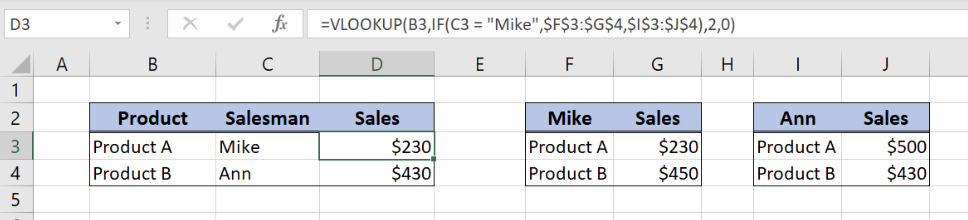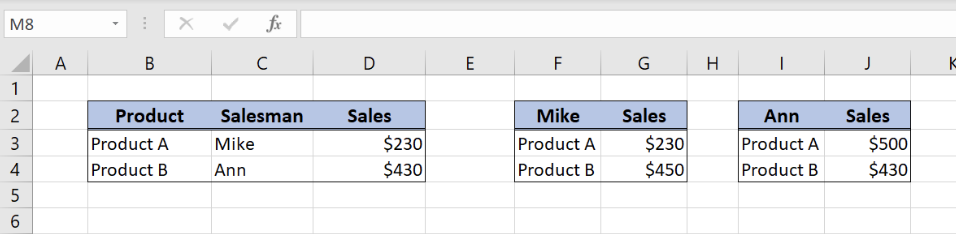Get instant live expert help with Excel or Google Sheets“My Excelchat expert helped me in less than 20 minutes, saving me what would have been 5 hours of work!”

#### Post your problem and you’ll get Expert help in seconds.

Your message must be at least 40 characters
Our professional Expert are available now. Your privacy is guaranteed.

# Using VLOOKUP with Multiple Lookup Tables: Best Practices

While working in Excel, we often need to get values from multiple tables. This is possible by using VLOOKUP and IF functions. In this tutorial we will learn how to pull values from several tables, using VLOOKUP function with multiple lookup tables.Figure 1. Final result

## Syntax of the VLOOKUP formula with 2 tables

First, we will use the IF function to determine which table we will reference in our lookup. Then, we will nest the IF function inside the VLOOKUP function to create a dynamic lookup formula.

The generic formula to lookup from 2 tables looks like:

`=VLOOKUP(lookup_value, IF(logical_test, table1, table2), 2, 0)`

The parameters of the IF function are:

• logical_test – a logical test which will decide which table to use
• [value_if_true] – if a logical condition is true, table1 will be a result of IF function
• [value_if_false] – if a logical condition is false, table2 will be a result of IF function.

The parameters of the VLOOKUP function are:

• lookup_value – a value which we want to find in a lookup table
• table_array: IF(logical_test, table1, table2) – a table in which we want to look up. The table will be a result of IF function, depending on a condition
• col_index_num – a column number in lookup table which value we want to pull
• range_lookup – default value 0. This means that we want to find an exact match for a lookup value.Figure 2. Tables for VLOOKUP

We will now look at the example to explain in details how this function works. Above all, let’s start with examining the structure of the data that we will use.

The first table consists of 3 columns:

• The first column is “Product” (Column B)
• The second one is “Salesman” (Column C),
• While the third one is “Sales” (Column D).

The second and third tables have data about Mike’s and Ann’s sales per product.:

• Columns F and G have products and sales for Mike,
• Columns I and J have products and sales for Ann.

As a result, we want to get sales value in the first table (column D), depending on salesman and product.

## Get Product Sales Value from SalesmanFigure 3. Using VLOOKUP with multiple lookup tables

The formula looks like:

`=VLOOKUP(B3,IF(C3 = "Mike",\$F\$3:\$G\$4,\$I\$3:\$J\$4),2,0)`

In our example, lookup_value is “Product” column (B3 cell), while the table_array depends on “Salesman” column value. Therefore, if the salesman is Mike, we will look up in the second table (F3:G4).

On the other hand, if the salesman is not Mike, we will lookup in the third table (I3:J4). Furthermore, the IF function will return a table_array parameter for VLOOKUP function. Col_index_num has value 2, as we want to pull value from the second column of the range. Finally, range_lookup has value 0, because we want to find an exact match of “Product” values.

To use VLOOKUP with 2 tables, we need to follow these steps:

• Select cell D3 and click on it
• Insert the formula: `=VLOOKUP(B3,IF(C3 = "Mike",\$F\$3:\$G\$4,\$I\$3:\$J\$4),2,0)`
• Press enter
• Drag the formula down to the other cells in the column by clicking and dragging the little “+” icon at the bottom-right of the cell.

As a result, we will get sales \$230 in the cell D3. The value of “Salesman” in C3 is Mike, so the IF function will return first table (F3:G4) as a lookup table. Finally, the VLOOKUP will return \$230, as the product is Product A in the cell B3.

Most of the time, the problem you will need to solve will be more complex than a simple application of a formula or function. If you want to save hours of research and frustration, try our live Excelchat service! Our Excel Experts are available 24/7 to answer any Excel question you may have. We guarantee a connection within 30 seconds and a customized solution within 20 minutes.

Are you still looking for help with the VLOOKUP function? View our comprehensive round-up of VLOOKUP function tutorials here.

### Did this post not answer your question? Get a solution from connecting with the expert.Another blog reader asked this question today on Excelchat:
Solution exampleshow do i create a formula to change a number to a line of text, ie i have about 20 descriptions of tasks on a timesheet, and instead of repeatedly typing text i was wondering if i could create a short cut.. ie. type the number 1 or a short cut and a line of text appears in the cell.. for example task 1 : meeting on site to discuss measurements. task 2: meeting in house with MD to discuss progress and so on
Solved by S. D. in 58 minsHi. If i want to write a formula that reverts a scale in example column B, to the opposite in column C. Ex. all value 5 to 1, all value 4 to 2, all value 3 to 3, all value 2 to 4 and all value 1 to 5. Is it possible to use the IF function, or another function to do this?
Solved by V. A. in 18 minsI need help with a vlookup for multiple duplicate references
Solved by M. D. in 16 minsI require a name on one field to match a name ('DATA'!AL17), to those on another sheet, within a range of names (SUBURBS!\$A2:\$A1659), which also contains fields of demographic data, if the names match, return one of the data sets within that range (the average income of that suburb). This formula currently returns \$0.00 =IF(SUBURBS!\$A2:\$A1659='DATA'!AL17,SUBURBS!\$G2:\$G1659,0)
Solved by D. D. in 10 minsi want to string @ if together to turn week number to month. so 1 to 4 = "JAN" .. 5 to 9 = "FEB" .. having trouble connecting the @ if together
Solved by T. B. in 32 mins## Subscribe to Excelchat.coAnother blog reader asked this question today on Excelchat: